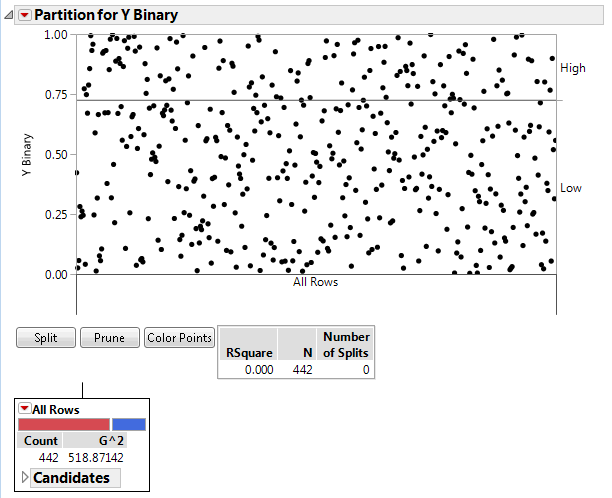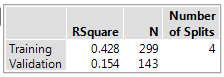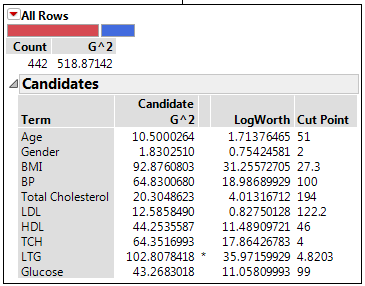Publication date: 11/10/2021

## Report for Categorical Responses

The sample data table Diabetes.jmp was used to create a report for the categorical response Y Binary.

Figure 4.6 Partition Report for a Categorical Response### Partition Plot

Each point in the Partition Plot represents an observation in the data table. If validation is used, the plot is only for the training data. The initial partition plot does not show splits.

Notice the following:

The left vertical axis is the proportion of each response outcome.

The right vertical axis shows the order in which the response levels are plotted.

Horizontal lines divide each split by the response variable. The initial horizontal line shows the overall proportion of the first plotted response in the data set.

Splits are shown below the X axis with a text description and a vertical line that splits the observations in the plot. The vertical lines extend into the plot and indicate the boundaries for each node. The most recent split appears directly below the horizontal axis and on top of existing splits. The plot is updated with each split or prune of the decision tree.

### Summary Report

Figure 4.7 Summary Report for a Categorical ResponseThe Summary Report provides fit statistics for the training data and validation and test data (if used). The fit statistics in the Summary Panel update as you add splits or prune the decision tree.

RSquare

The current value of R2.

N

The number of observations.

Number of Splits

The current number of splits in the decision tree.

### Node Reports

Each node in the tree has a report and a red triangle menu with additional options. Terminal nodes also have a Candidates report.

Figure 4.8 Terminal Node Report for a Categorical ResponseCount

Number of training observations that are characterized by the node.

G2

A fit statistic used for categorical responses (instead of sum of squares that is used for continuous responses). Lower values indicate a better fit. See Statistical Details for the Partition Platform.

Candidates

For each column, the Candidates report provides details about the optimal split for that column. The optimal split over all terms is marked with an asterisk.

Term

Shows the candidate columns.

Candidate G^2

Likelihood ratio chi-square for the best split. Splitting on the predictor with the largest G^2 maximizes the reduction in the model G^2.

LogWorth

The LogWorth statistic, defined as -log10(p-value). The optimal split is the one that maximizes the LogWorth. See Statistical Details for the Partition Platform.

Cut Point

The value of the predictor that determines the split. For a categorical term, the levels in the left-most split are listed.

The optimal split is noted by an asterisk. However, there are cases where the Candidate G2 is higher for one variable, but the Logworth is higher for a different variable. In this case > and < are used to point in the best direction for each variable. The asterisk corresponds to the condition where they agree. See Statistical Details for the Partition Platform.